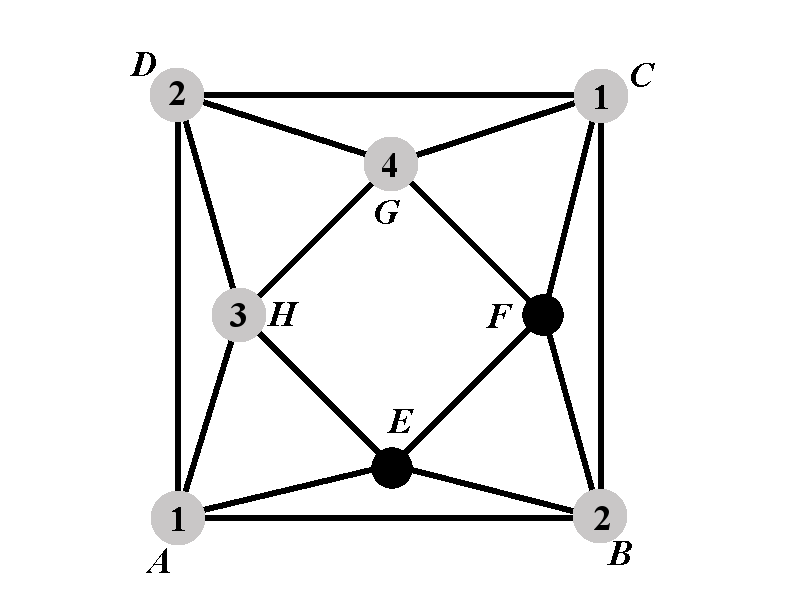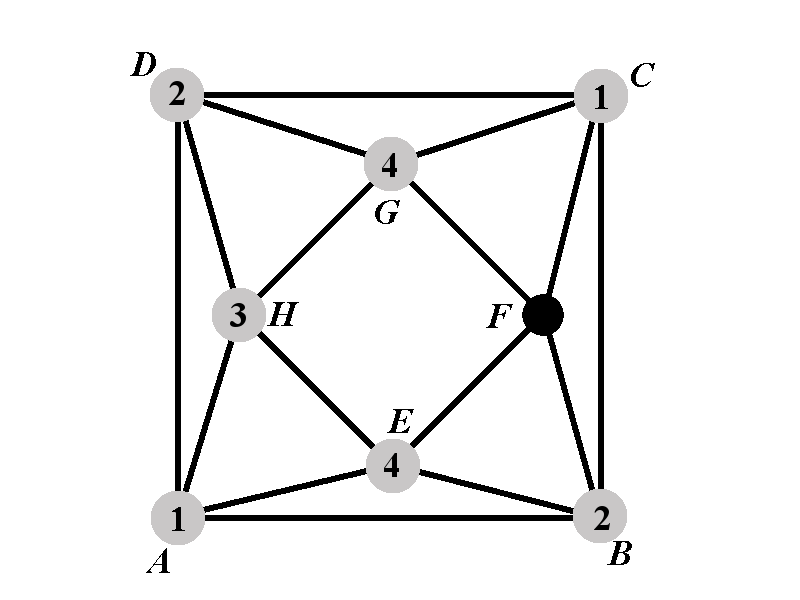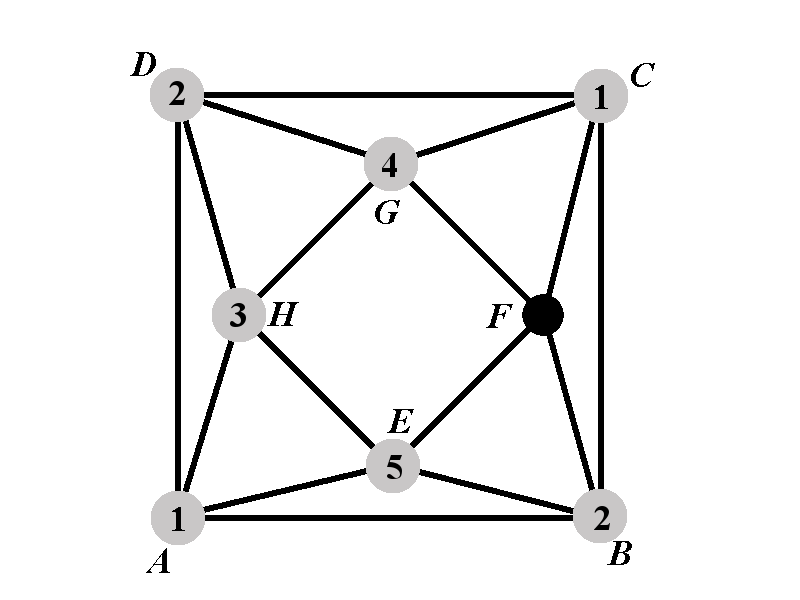Back to: Chromatic Polynomial
##### Case 1.2: Calculation of $$P_{1.2^{\circ}}(k)$$The vertex $$E$$ cannot have a color from $$\{1,2,3\}$$. We will consider two sub-cases. In the sub-case 1.2.1 we assume that the vertex $$E$$ has the color $$4$$. In the sub-case 1.2.2 we assume that the vertex $$E$$ has one of the $$(k-4)$$ colors outside of the set $$\{1,2,3,4\}$$. Let us denote by $$P_{1.2.1^{\circ}}$$ the number of proper colorings in which the vertices $$A$$, $$B$$, $$C$$, $$D$$, $$E$$, $$G$$, and $$H$$ have the colors $$1$$, $$2$$, $$1$$, $$1$$, $$4$$, $$4$$, and $$3$$, repsectively. Denote by $$P_{1.2.2^{\circ}}(k)$$ the number of proper colorings in which the vertices $$A$$, $$B$$, $$C$$, $$D$$, $$E$$, $$G$$, and $$H$$ have the colors $$1$$, $$2$$, $$1$$, $$2$$, $$5$$, $$4$$, and $$3$$, respectively.

The number $$P_{1.2^{\circ}}(k)$$ can be expressed in terms of these newly introduced numbers as $P_{1.2^{\circ}}(k)=P_{1.2.1^{\circ}}(k)+(k-4)P_{1.2.2^{\circ}}(k).$

## Case 1.2.1: Calculation of $$P_{1.2.1^{\circ}}(k)$$It remains to count the number of colorings for the vertex $$F$$. The vertex $$F$$ can have any color outside of $$\{1,2,4\}$$. There are $$k-3$$ such colors, hence $P_{1.2.1^{\circ}}(k)=k-3.$

##### Case 1.2.2: Calculation of $$P_{1.2.2^{\circ}}(k)$$The vertex $$F$$ can have any color outside of the set $$\{1,2,4,5\}$$. There are $$k-4$$ available colors for $$F$$ hence $P_{1.2.2^{\circ}}(k)=k-4.$

##### Finalizing the Calculation of $$P_{1.2^{\circ}}(k)$$

Using that $$P_{1.2^{\circ}}(k)=P_{1.2.1^{\circ}}(k)+(k-4)P_{1.2.2^{\circ}}(k)$$, $$P_{1.2.1^{\circ}}(k)=k-3$$, and $$P_{1.2.2^{\circ}}(k)=k-4$$, we obtain $P_{1.2^{\circ}}(k)=k-3+(k-4)^2.$